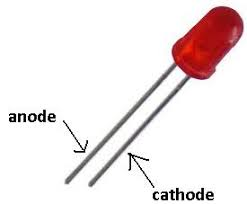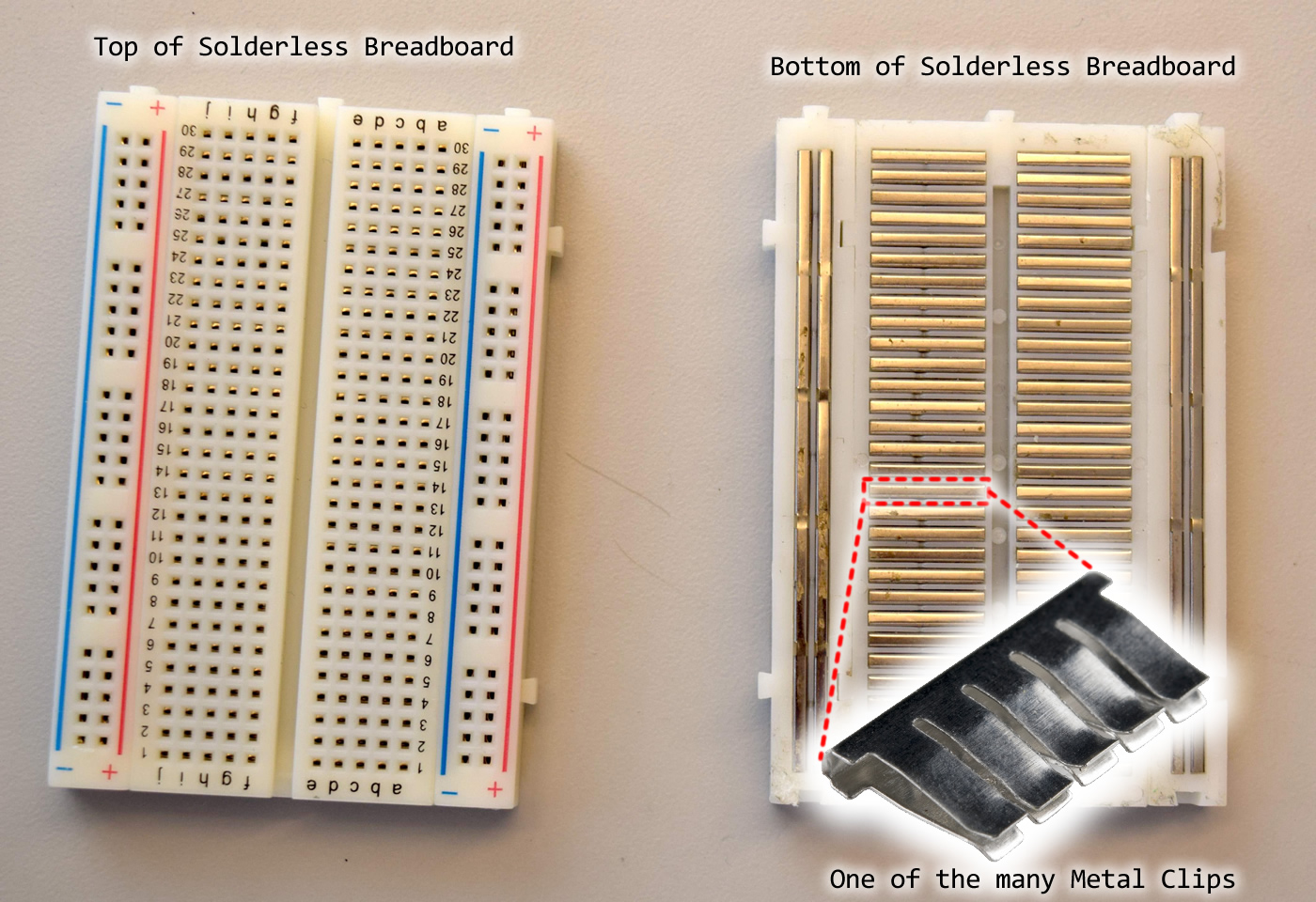Controlling Traffic Lights With Arduino Uno

Controlling Traffic Lights With Arduino Uno

Welcome to our project of building automatically controlled traffic lights.

Materials:

Red LED                                 x 1
Yellow LED                             x 1
Green LED                              x 1
220Ω resistor                         x 3
Arduino-Genuino Uno           x 1
Male to Male jumper wires    x 4

Explanation of Materials:

Resistor:

A resistor is used to decrease the current power for the led. There are many type of resistors. We differentiate by their colour codes .The diagram given on the right is just given for your reference.Led

As shown in the image on the left

The long terminal which is the anode is the positive terminal of the led.

The short terminal which is the cathode is the negitive terminal of the led.

Arduino-Genuino Uno

The parts of the arduino uno are given .

The pins at the right of the board from 13 to 2 are output and input pins .

The 1 and 0 pins are the TX and RX pins

The upper left pins are the 5v, GND and other pins.The solderless breadboard is ideal for testing projects.The diagram is given:

At the bottom of the breadboard, the sides of the breadboard are connected together There are 1 positive and 1 negative rails on each side.

In the between each row has 5 pins connected to each other.

For the explanation video, see:

In this video I have shown the function of the parts i have used in this project.

Connections:

Now we know the function of each part, let’s talk about the connections:

Pin 8 is of Arduino connected to the red led’s positive

Pin 10 of Arduino is connected to the yellow led’s positive

Pin 12 of arduino is connected to the green led’s positive

GND of arduino is connected to the negative rail of the breadboard

All the negative pins of led are connected to the negative rail of the breadboard.Connection Explanaton Video:

In this video I have explained how the connections work in project

Code:

The code is given at the end of the page or download it.

Code explanation:

#define Red_pin 8
#define Yellow_pin 10
#define Green_pin 12

The #define function defines the

‘1 parameter’ Red_pin as the ‘2 parameter’ 8.

We can’t change the no. by adding as it is a constant.

void setup() {
// initialize digital pin 13 as an output.
pinMode(Red_pin, OUTPUT);
pinMode(Yellow_pin, OUTPUT);
pinMode(Green_pin, OUTPUT);

}

The void setup function runs the code only once.

The pinMode(Red_pin, OUTPUT) inside the void setup function defines the pin no. which is the first parameter and the function that is the output which is the second parameter.

void loop() {
digitalWrite(Red_pin, HIGH);
delay(3000);
digitalWrite(Yellow_pin, HIGH);
delay(1000);
digitalWrite(Red_pin, LOW);
digitalWrite(Yellow_pin, LOW);
digitalWrite(Green_pin, HIGH);
delay(3000);

The void loop function runs the program forever. We have to upload another program to stop it . The digitalWrite function switches off and on the given pin no. In digitalWrite(Red_pin, HIGH);  the 1st parameter is the pin no we want to output to and the 2nd parameter is the state of the pin. HIGH/1 is on and LOW/0 is off. The delay function stops the program for the given milliseconds.

Code Explanation Video:

Testing:

Now we have understood the code and the connections and code, so let’s test our project